﻿ Simulation of Material Behaviour by Bodner-Partom Model

### Simulation of Material Behaviour by Bodner-Partom Model

Jozef Bocko, Viera Nohajová, Juraj Šarloši

American Journal of Mechanical Engineering

## Simulation of Material Behaviour by Bodner-Partom Model

Jozef Bocko1,, Viera Nohajová1, Juraj Šarloši1

1Department of Applied Mechanics and Mechanical Engineering, Faculty of Mechanical Engineering, Technical University of Košice, Košice, Slovak Republic

### Abstract

The paper deals with application of Bodner-Partom material model for description of material behavior. The results from experimental measurements of test specimens of given material were used for identification of parameter of Bodner-Partom model by using procedures based on genetic algorithms. The parameters of material were then used in computations by procedure UMAT of finite element program ABAQUS. The results of computations demonstrate ability of model to simulate material behavior with satisfied accuracy.

• Jozef Bocko, Viera Nohajová, Juraj Šarloši. Simulation of Material Behaviour by Bodner-Partom Model. American Journal of Mechanical Engineering. Vol. 3, No. 6, 2015, pp 181-185. http://pubs.sciepub.com/ajme/3/6/5
• Bocko, Jozef, Viera Nohajová, and Juraj Šarloši. "Simulation of Material Behaviour by Bodner-Partom Model." American Journal of Mechanical Engineering 3.6 (2015): 181-185.
• Bocko, J. , Nohajová, V. , & Šarloši, J. (2015). Simulation of Material Behaviour by Bodner-Partom Model. American Journal of Mechanical Engineering, 3(6), 181-185.
• Bocko, Jozef, Viera Nohajová, and Juraj Šarloši. "Simulation of Material Behaviour by Bodner-Partom Model." American Journal of Mechanical Engineering 3, no. 6 (2015): 181-185.

 Import into BibTeX Import into EndNote Import into RefMan Import into RefWorks

123
Prev Next

### 1. Introduction

Simulation of stress and deformation states of mechanical system is connected with description of material behaviour. Many different material models were developed during the history of continuum mechanics. The simplest models that are based on Hooke law of linear dependency of stresses on strains are usable only in case of small deformations and simple materials. In practice is often necessary to describe complex material behaviour that include plastic or visco-plastic behaviour. Different models have been developed for such simulations and many from those are also included in commercial finite element codes. In the paper we describe application of Bodner-Partom model for simulation of behaviour of aluminium alloy. The parameters of model were identified from experimental measurements by procedures based on minimization of differences between measured and computed results by genetic algorithm . The genetic algorithms use the principles created and used in nature and they are often applied in common engineering practice.

At the end of paper are given graphs representing ability of Bodner-Partom model to represent measured response of test specimens to loading.

### 2. Theoretical Background

2.1. Bodner-Partom Material Model

The Bodner-Partom material model consists of a system of constitutional equations for simulation of behaviour of visco-elasto-plastic material under small deformation and arbitrary loading history. 8 parameters of model can be identified from the tensile tests [5-12,14,15].

The model uses additive splitting of strains or strain rates into elastic and plastic parts. Accordingly strain rate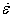has two additive components: elastic rate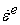and plastic rate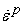.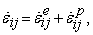(1)

where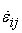is total strain rate and both their components are in general not equal to zero in different conditions of loading or unloading. Elastic strain rate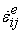represents elastic velocity of deformation related to stress rate by relation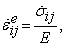(2)

where E is Young’s modulus of elasticity.

Rate of plastic strain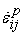is considered to be a function of actual state quantities, especially stress, represented by components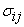of stress tensor, internal state quantities depending on load history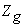, total strain ratea  temperature T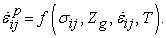(3)

The parametersthat depend on history loading represents hardening of material during plastic deformation andis total strain rate. For small deformations we can ignore dependency of plastic strain rateon total strain rate.

Effective values of strain rate are computed from relation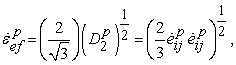(4)

and effective stress from relation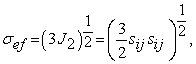(5)

where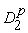is a scalar invariant of deviator of plastic strain rate,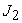is a second scalar invariant of stress deviator,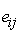represents components of deviator of strain tensor,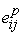represents components of deviator of plastic strain and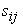are components of deviator of stress tensor.

The plastic strain rate is very small in case of small stress and in case of high stress it has a limited saturation ability. Evolution of deformation is based on equation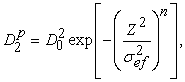(6)

where n is a parameter that is connected with sensitivity to velocity, Z represents a scalar parameter of hardening that depends on the history of loading, i.e. on internal state quantities that can contain isotropic or directional components.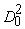is a limit value of. Both, n as well as Z depends in general on temperature. Equation (6), represents general macroscopic description of plastic reaction to dislocation mechanisms activated by thermal processes.

The general relation for components of plastic strain rate is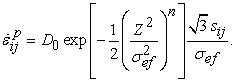(7)

Accordingly, in case of one-dimensional loading resulting to stress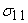and simple shear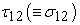we have relations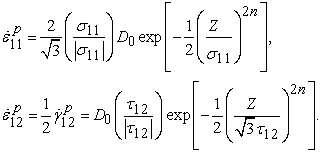(8)

Here,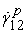is a rate of plastic shear strain and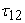is shear stress.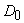represents a limit value of plastic strain rate in shear in case of high shear stress or a hardening parameter Z tends to be zero.

Increasing Z leads to increasing of resistance against plastic flow. The hardening parameter Z consists of two components: component of isotropic hardening Z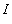and directional hardening Z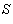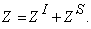(9)

The component of isotropic hardening is expressed by relation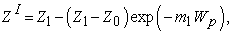(10)

where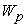is accumulated plastic work with respect to unit volume starting with zero for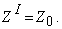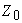and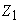are starting and maximal value of isotropic hardening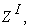respectively.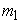is a quantity that regulates velocity of hardening.

The component of directional hardening is expressed by equation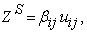(11)

whererepresents directional cosines of actual stress state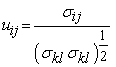(12)

and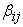represents a variable of directional hardening.

Further we will consider case of one-dimensional loading with isotropic and directional hardening without temperature influence. This special integration procedure was accomplished in Matlab .

In the model are not applied loading and unloading criterion, nor surface of plasticity and the plastic deformation is nonzero in all states of loadings. The material constants that can be determined from Bodner-Partom model are: parameter of regulation of plastic strain rate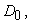sensitivity parameter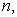starting value of isotropic hardening, maximal value of isotropic hardening, parameter controlling velocity of isotropic hardening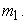These values together with Young’s modulus E, shear modulus G and Poisson ratio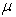are input values for the Bodner-Partom model. All parameters are evaluated from tensile tests.

2.2. Genetic Algorithm

The identification of Bodner-Partom material model was accomplished by genetic algorithm. The genetic algorithm belongs to the group of so-called evolution algorithms. Evolution algorithms are divided to the three categories of programs:

•  evolution strategy,

•  evolution programming,

•  genetic algorithm.

Evolution algorithm is a searching algorithm over certain population. It takes into account several candidates for optimal solution. Every candidate is an individual and it is a part of population. From the starting population are chosen the best individuals that fulfil certain criterion and those serve as a base for creation of next generations. The parameters of given model are transformed to the chromosomes and by operations of genetic algorithms they are used for optimization. In our case we have used the following parameter of Bodner-Partom model: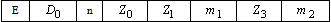The aim of optimization was to find the best parameters of model that approximate results of experimental measurements realized on test specimens. The objective function represented squares of differences between curves gained from measurements and those computed by numerical procedures for one-dimensional model.

### 3. Measurement and Parameter Identification

3.1. Experimental Measurement

The measurements were realized with test specimens made of aluminium alloy of unknown quality with shape and dimensions given by Standard STN EN 10002-1, Figure 1. The test specimens were loaded by one-dimensional tensile force on the tensile machine WILLE GEOTECHNIK, Figure 2 and Figure 3.

The specimens were loaded by continuously increased force resulting to different deformation velocity and the results were represented by pairs of values stress – strain.Download asVeiw figureFigures index
Figure 1. Dimension and shape of test specimenDownload asVeiw figureFigures index
Figure 2. Tensile test machine

Three measurements were realized with three specimens. Every specimen was loaded with different velocity of elongation – specimen 1 with velocity 0.25 mm/min, specimen 2 with velocity 1 mm/min and specimen 3 with velocity 4 mm/min.Download asVeiw figureFigures index
Figure 3. Detail of specimen fixation
3.2. Parameter Identification

Program for searching the best parameters of Bodner-Partom model works on the principle of algorithm which starts with initialization of the population of zero generation. There are generated individuals that represents 8 parameters of Bodner-Partom model in the zero generation. Next, the individuals are evaluated and they enter the evolution cycle for the solution of problem (solution of constitutive equations of model in question and approximation of curve gained from the model and measured tensile loading diagram). If the variables of genetic algorithm fulfil stop condition, the algorithm stops and the last best individual is used for solution. In case the stop condition is not fulfilled, then new generation is created from the actual one and the algorithms continues.

The genetic algorithm was used for every specimen with its own prescribed deformation velocity. In the following is given an example for the first specimen, where the genetic algorithm used:

•  24 individual in population,

•  maximal number of generation was set up from 100 to 300,

•  residual stochastic method applied for crossing,

•  scattering method for crossing,

•  number of elite individuals was 8, which is 33,33% of whole number of individuals of population.

The control parameters significantly influence the genetic algorithm program so that suitable selection of those parameters can improve its running. It have to be said that there is no ensured repeatability of results, because the program is based on stochastic random number generators leading to different results. In Figure 4 is given a stress – strain relation for the best parameters gained by approximation of measured and computed curve using genetic algorithm for the specimen 1.Download asVeiw figureFigures index
Figure 4. Stress-strain relations for the specimen 1

These results were reached for 50 individuals in population, 300 generations. The total time of computation was 13 059 seconds. Resulting parameters for Bodner-Partom material model are given in Table 1.

#### Table 1. Parameters of Bodner-Partom ModelDownload asPowerPoint Slide

Veiw figure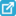View current table in a new window

### 4. Application of Parameters in FEM

The parameters of Bodner-Partom model computed by program Matlab were further used in finite element program ABAQUS [1, 2, 3]. For the computation was used subroutine UMAT. The subroutine UMAT is necessary for realization of computational material models that are not included in program ABAQUS.Download asVeiw figureFigures index
Figure 5. Model of test specimen

The equations of Bodner-Partom material model were written in program FORTRAN and implemented into ABAQUS as material subroutine UMAT for isotropic visco-elasto-plastic material with isotropic and kinematic hardening without thermal influences . The program was compiled and linked to the ABAQUS and the all preprocessing as well as postprocessing abilities of this program are usable for simulations.

The parameter values given in Table 1 were applied to the three-dimensional model for classic tensile test the shape and dimension of which are given in Figure 1. The 3D finite element model is given in Figure 5 and the mesh of finite elements is shown in Figure 6.Download asVeiw figureFigures index
Figure 6. Mesh of finite elements

An example of von Mises stress field in the specimen 1 is given in Figure 7.Download asVeiw figureFigures index
Figure 7. Equivalent von Mises stresses in specimen 1

The stress – strain relations computed by program ABAQUS for all three specimens are given in Figure 8.Download asVeiw figureFigures index
Figure 8. Computed stress – strain relations

The results of computed stress – strain charts enriched by two new deformation velocities 2 mm/min and 8 mm/min are given in Figure 9.Download asVeiw figureFigures index
Figure 9. Computed stress – strain relations for measured and new different deformation velocities

### 5. Conclusions

The paper is devoted to modelling of material properties in the area of elasto-visco-plastic deformation by Bodner-Partom material model. The modelling was based on experimental measurement of stress – strain curves for different velocities of deformation and the identification of parameters was accomplished by optimization method based on genetic algorithm.

It was found out that genetic algorithms are sensitive to input control data so that it is suitable to repeat the computations with different inputs in order to reach optimal values for curve approximations. Some parameters that are used implicitly can be optimal, but in general it is good to change different values. It means, experience is necessary for application this optimization procedure. In general it is good to use big number of individuals in generation as well as huge number of generations if we want to come to optimal results. However, high values of those parameters lead to time-consuming run of program. In our case we have found as optimal 300 generations each with 24 individuals.

We have applied those parameters to several specimens made of elasto-visco-plastic materials loaded with prescribed deformation velocities. Additional computation were realized also for different deformation velocities.

The results from tensile test measurements and parameter identifications were used by program UMAT, which allows use specific material models not included in standard ABAQUS material library. The program was applied for simulation of visco-elasto-plastic material properties of aluminium alloy specimens. The results of measurements and computations show very good agreement and accordingly all steps of above mentioned process that include measurement, parameter identification and computation by the finite element programs are correct.

### Acknowledgement

This article was created with support of VEGA grant projects VEGA 1/1205/12 Numerical modelling of mechatronic systems.

### References

  “Abaqus Documentation, ” Available: http://abaqusdoc.ucalgary.caIn article  “Abaqus Tutorial, ” Available: http://abaqus.me.chalmers.seIn article  “Abaqus UMAT Implementation, ” Available: http://www.ifb.ethz.ch/education/masterconstitutivemodeling/fem.In article  Bocko, J., Müller, J., “Evolutionary procedures in structural optimization,” Transactions of the University of Košice, 75-82, 1999.In article  Bodner, S.R., Unified Plasticity for Engineering Applications. New York: Kluwer Academic, Plenum Publishers, 2002.In article View Article  Bodner, S.R., Aboudi, J., “Stress wave propagation in rods of elastic visco-plastic material,” Solid Structures, 19 (1). 305-31, 1983.In article View Article  Bodner, S.R., Chan, K.S., “Modeling of continuum damage for application in elasto-viscoplastic constitutive equations,” Engineering Fracture Mechanics, 25, 705-712, 1986.In article View Article  Bodner, S.R., Naveh, M., Viscoplastic Shell Buckling. Buckling of Structures, Amsterdam: Elsevier Science Publishers, 1988.In article PubMed  Bodner, S.R., Partom, Y., “Constitutive Equations for Elastic-Viscoplastic Strain Hardening Materials,” ASME, 42 (2). 385-389, 1975.In article  Bodner, S.R., Rubin, M.B., “An incremental elasto-viscoplastic theory indicating a reduced modulus for non-proportional buckling,” Solid Structures, 32 (20). 2967- 2987, 1995.In article View Article  Krausz, A.S., Constitutive Laws of Plastic Deformations. New Yourk: Academic Press, 1996.In article PubMed  Miller, A.K., Unifield constitutive Equations for Plastic Deformation and Creep of Engineering Alloys, New York: Elsevier Applied Science, 1987.In article View Article  Qi, W., Brocks, W., ABAQUS user subroutines for the simulation of viscoplastic behaviour including anisotropic damage for isotropic materials and for single crystals. Technical Note GKSS/WMS/01/5, Berlin, 2001.In article  Pyrz, M., Parametres Identification of Viscoplastic Models Using Evolutionary Algorithms. XXI ICTAM, Warsaw, 2004.In article PubMed  Sansour, C., Bocko, J., On the numerical implications of multiplicative inelasticity with an anisotropic elastic constitutive law, International Journal of Numerical Methods in Engineering, 58 (14). 2131-2160, 2003.In article View Article